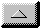Publications Department

Doppler Correction for Interstellar Communications
by Marko Cebokli, S57UUU (email s57uuu@hamradio.si)

Digital signal processing, such as is used for SETI, divides a wide received spectrum into a large number of narrow bins, and requires that we integrate the signals within these bins for long periods of time. But because of the Doppler shift caused by our Earth's motion through space, the received signal tends to be smeared between adjacent bins, and lost in the noise. Thus, it is common practice to calculate real-time Doppler shift, and to compensate for it at the receive end, in either hardware or in software.

One popular technique involves slowly tuning the local oscillator over time (a process known as dechirping), to compensate for the expected rate of change in frequency of the received signal. In doing so, we usually choose the Galactic center as our reference point of rest. One presumes that a deliberately beamed interstellar beacon will be similarly frequency-compensated relative to the Galactic center of rest, to give the transmitter and the receiver a common frequency reference, maximizing chances of detection.

It is not hard to calculate the Doppler shift for a given relative motion. For speeds small compared to c (light speed) you can use the linear approximation: fractional frequency shift = fractional c speed. For example, if you are traveling at 1% of c, your frequency will be 1% off.

Most of the Doppler chirp we observe in radio astronomy is generated by the Earth's rotation on its axis. The tangential Earth rotation speed at the equator is roughly 40000 km in 86163 sec (one sidereal day), or 0.46 km/sec or 1.54E-6c. If you're not on the equator, multiply this speed by the cosine of your latitude. Also, if you're tracking a star that is not on the celestial equator, you again multiply the speed with the cosine of the declination of that star.

Because the distance between transmitter and receiver is much greater than the Earth's diameter, the Doppler curve will be a simple 24-hour sinusoid (23h 56m 03s exactly). Its amplitude is determined by the above calculated speed, and its phase is such that it is positive when the star rises, and goes through zero when the star transits your meridian. Therefore, its slope is always negative while the star is above the horizon. (This is strictly true only on the equator. For example for circumpolar stars at a higher latitude, you can see the whole curve, up and down.) For example, you're at 40 deg N and track a star at 20 deg N with a 1400 MHz receiver. The maximum relative speed caused by the Earth's rotation is 1.54E-6 * 0.77 * 0.94 = 1.11E-6 times the light speed. Thus the amplitude of the Doppler curve is 1400E+6 * 1.11E-6 = 1554Hz. The angular frequency (omega) of Earth rotation is 2*Pi/86400 or 7.3E-5 rad/sec. So your Doppler frequency offset curve is 1554*sin(7.3E-5 * t) Hz, with t counted in seconds and zero at the moment your target star transits your meridian (azimuth of your dish due south, for Northern hemisphere observers).

To see how long you can integrate without dechirping, you need to know the maximum slope of the frequency curve. The slope peaks when the sine crosses zero and for a*sin(b*t) equals a*b, or 0.11 Hz/sec (square Hz) for the above example. If we use 10 Hz bins, the maximum non-dechirped integration time would be 90 sec (a minute and a half). This maximum chirp happens when the star transits, so you could use somewhat longer integration times when the star rises/sets. Also, pointing closer to celestial pole (and moving closer to Earth's poles) will allow you to use longer times.

For the contribution of the Earth's orbital revolution around the Sun, the exact calculation is more complicated because the orbit is not a circle. But the eccentricity is only a few percent, so a good approximation is possible using a circular orbit. The speed here is 9.42E8 km in 31.6E6 seconds, or 29.8 km/s, or approximately 1E-4c. This is the maximum speed; you must multiply it by the cosine of the target star's declination in ecliptic coordinates. For 1.4 GHz, the worst case Doppler is 140 kHz. The omega of once per year is approx 2E-7 rad/sec. The (max) Doppler curve is 1.4E5 * sin (2E-7 * t) Hz, the phase and amplitude being determined by the star's ecliptic coordinates. It does not depend on your location on Earth.

The maximum slope of this curve is 0.028Hz/sec. Thus, for the worst case (tracking a star on the ecliptic in a direction perpendicular to Earth's travel - that means looking towards the Sun or the anitsolar point), your maximum integration time if you dechirp only for Earth rotation is about 6 minutes. That's the worst case though, so 30 min integration with 10Hz bins would be quite reasonable, provided you dechirp at least the Earth's rotation. The Sun's travel around the Milky way has such a long period that for any practical purpose it only adds a constant frequency shift (that depends on galactic coordinates in this case) which doesn't influence the possible integration time.

In conclusion, these guidelines apply to integration limits for our typical Project Argus station (10Hz bins):

• We can integrate for tens of seconds with no Doppler dechirping.
• We can integrate for tens of minutes by compensating for Earth rotation Doppler only.
• For greater integration times, we must also compensate for the Doppler effects of the Earth's orbit around the Sun.
• We never have to dechirp for the Sun's motion around the Galactic center (at least, not for integration times consistent with a human lifespan!)email theWebmaster | Home | General | Memb Svcs | Publications | Press | Technical | Internet | Index | entire website copyright © The SETI League, Inc. this page last updated 28 December 2002Top of Page